## C# || How To Get The Number Of Binary Tree Paths Equal To Path Sum Using C#The following is a module with functions which demonstrates how to get the number of binary tree paths equal to path sum using C#.

1. Number Of Path Sum Paths – Problem Statement

Given the root of a binary tree and an integer targetSum, return the number of paths where the sum of the values along the path equals targetSum.

The path does not need to start or end at the root or a leaf, but it must go downwards (i.e., traveling only from parent nodes to child nodes).

Example 1: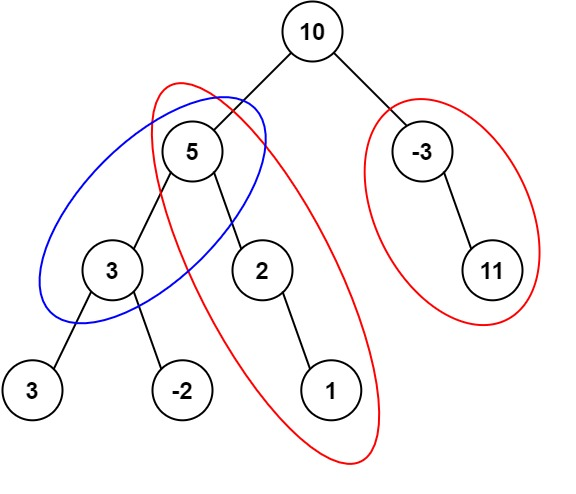``` Input: root = [10,5,-3,3,2,null,11,3,-2,null,1], targetSum = 8 Output: 3 Explanation: The paths that sum to 8 are shown. ```

Example 2:

``` Input: root = [5,4,8,11,null,13,4,7,2,null,null,5,1], targetSum = 22 Output: 3 ```

2. Number Of Path Sum Paths – Solution

The following is a solution which demonstrates how to get the number of binary tree paths equal to path sum.

The main idea here is that the sum at each level for each path is calculated. When the next level is explored, the value at the previous level is summed together with the node value at the current level.

A map dictionary is used to keep track of the sum at each level. If the prefix sum at the previous level is enough to equal the target path sum at the current level, the result count is incremented.

``` 2. Number Of Path Sum Paths - Solution C# // ============================================================================ // Author: Kenneth Perkins // Date: Oct 18, 2021 // Taken From: http://programmingnotes.org/ // File: Solution.cs // Description: Determines how to get the number of tree paths equal to path sum // ============================================================================ /** * Definition for a binary tree node. * public class TreeNode { * public int val; * public TreeNode left; * public TreeNode right; * public TreeNode(int val=0, TreeNode left=null, TreeNode right=null) { * this.val = val; * this.left = left; * this.right = right; * } * } */ public class Solution { private int result = 0; private Dictionary<int, int> sumFrequency = new Dictionary<int, int>(); public int PathSum(TreeNode root, int targetSum) { sumFrequency = 1; Search(root, targetSum, 0); return result; } public void Search(TreeNode node, int targetSum, int currentSum) { if (node == null) { return; } // Determine the current sum currentSum = currentSum + node.val; // Get the path prefix sum var prefixSum = currentSum - targetSum; if (sumFrequency.ContainsKey(prefixSum)) { result += sumFrequency[prefixSum]; } // Increment the number of times this prefix has been seen sumFrequency[currentSum] = (sumFrequency.ContainsKey(currentSum) ? sumFrequency[currentSum] : 0) + 1; // Keep exploring along branches finding the target sum Search(node.left, targetSum, currentSum); Search(node.right, targetSum, currentSum); // Remove value of this prefixSum (path's been explored) --sumFrequency[currentSum]; } }// http://programmingnotes.org/ 1234567891011121314151617181920212223242526272829303132333435363738394041424344454647484950515253545556 // ============================================================================//    Author: Kenneth Perkins//    Date:   Oct 18, 2021//    Taken From: http://programmingnotes.org///    File:  Solution.cs//    Description: Determines how to get the number of tree paths equal to path sum// ============================================================================/** * Definition for a binary tree node. * public class TreeNode { *     public int val; *     public TreeNode left; *     public TreeNode right; *     public TreeNode(int val=0, TreeNode left=null, TreeNode right=null) { *         this.val = val; *         this.left = left; *         this.right = right; *     } * } */public class Solution {    private int result = 0;    private Dictionary<int, int> sumFrequency = new Dictionary<int, int>();     public int PathSum(TreeNode root, int targetSum) {        sumFrequency = 1;        Search(root, targetSum, 0);        return result;    }     public void Search(TreeNode node, int targetSum, int currentSum) {        if (node == null) {            return;        }         // Determine the current sum        currentSum = currentSum + node.val;         // Get the path prefix sum        var prefixSum = currentSum - targetSum;         if (sumFrequency.ContainsKey(prefixSum)) {            result += sumFrequency[prefixSum];        }         // Increment the number of times this prefix has been seen        sumFrequency[currentSum] = (sumFrequency.ContainsKey(currentSum) ? sumFrequency[currentSum] : 0) + 1;         // Keep exploring along branches finding the target sum        Search(node.left, targetSum, currentSum);        Search(node.right, targetSum, currentSum);         // Remove value of this prefixSum (path's been explored)        --sumFrequency[currentSum];    }}// http://programmingnotes.org/ ```

QUICK NOTES:
The highlighted lines are sections of interest to look out for.

The code is heavily commented, so no further insight is necessary. If you have any questions, feel free to leave a comment below.

Once compiled, you should get this as your output for the example cases:

``` 3 3 ```

## C# || How To Get All Root To Leaf Binary Tree Paths Equal To Path Sum Using C#The following is a module with functions which demonstrates how to get all the root to leaf binary tree paths equal to path sum using C#.

1. Root To Leaf Path Sum – Problem Statement

Given the root of a binary tree and an integer targetSum, return all root-to-leaf paths where the sum of the node values in the path equals targetSum. Each path should be returned as a list of the node values, not node references.

A root-to-leaf path is a path starting from the root and ending at any leaf node. A leaf is a node with no children.

Example 1: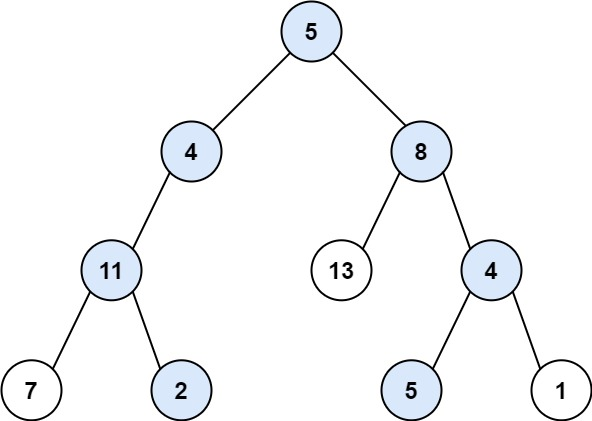``` Input: root = [5,4,8,11,null,13,4,7,2,null,null,5,1], targetSum = 22 Output: [[5,4,11,2],[5,8,4,5]] Explanation: There are two paths whose sum equals targetSum: 5 + 4 + 11 + 2 = 22 5 + 8 + 4 + 5 = 22 ```

Example 2: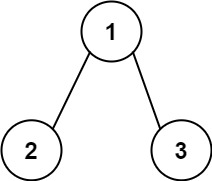``` Input: root = [1,2,3], targetSum = 5 Output: [] ```

Example 3:

``` Input: root = [1,2], targetSum = 0 Output: [] ```

2. Root To Leaf Path Sum – Solution

The following is a solution which demonstrates how to get all the root to leaf binary tree paths equal to path sum.

The main idea here is that the sum at each level for each path is calculated until we reach the end of the root-to-leaf.

A list is used to store the node value at each level. When the next level is explored, the value is appended to the list, and the process continues.

When we reach the end of the leaf, we check to see if the target value has been reached. If so, we add the node values that make up the path to the result list.

``` 2. Root To Leaf Path Sum - Solution C# // ============================================================================ // Author: Kenneth Perkins // Date: Oct 18, 2021 // Taken From: http://programmingnotes.org/ // File: Solution.cs // Description: Determines how to get all root to leaf path sum in a tree // ============================================================================ /** * Definition for a binary tree node. * public class TreeNode { * public int val; * public TreeNode left; * public TreeNode right; * public TreeNode(int val=0, TreeNode left=null, TreeNode right=null) { * this.val = val; * this.left = left; * this.right = right; * } * } */ public class Solution { private List<IList<int>> result = new List<IList<int>>(); public IList<IList<int>> PathSum(TreeNode root, int targetSum) { Search(root, targetSum, 0, new List<int>()); return result; } private void Search(TreeNode node, int targetSum, int currentSum, List<int> path) { if (node == null) { return; } // Add the node to this path path.Add(node.val); // Add the current value to the running total currentSum = currentSum + node.val; // Since this is a root-to-leaf check, evaluate for the // success condition when both left and right nodes are null if (node.left == null && node.right == null) { // Check to see if current value equals target if (currentSum == targetSum) { result.Add(new List<int>(path)); } } // Keep exploring along branches finding the target sum Search(node.left, targetSum, currentSum, path); Search(node.right, targetSum, currentSum, path); // Remove the last item added as this path has already been explored path.RemoveAt(path.Count - 1); } }// http://programmingnotes.org/ 123456789101112131415161718192021222324252627282930313233343536373839404142434445464748495051525354555657 // ============================================================================//    Author: Kenneth Perkins//    Date:   Oct 18, 2021//    Taken From: http://programmingnotes.org///    File:  Solution.cs//    Description: Determines how to get all root to leaf path sum in a tree// ============================================================================/** * Definition for a binary tree node. * public class TreeNode { *     public int val; *     public TreeNode left; *     public TreeNode right; *     public TreeNode(int val=0, TreeNode left=null, TreeNode right=null) { *         this.val = val; *         this.left = left; *         this.right = right; *     } * } */public class Solution {    private List<IList<int>> result = new List<IList<int>>();     public IList<IList<int>> PathSum(TreeNode root, int targetSum) {        Search(root, targetSum, 0, new List<int>());        return result;    }     private void Search(TreeNode node, int targetSum, int currentSum, List<int> path) {        if (node == null) {            return;        }         // Add the node to this path        path.Add(node.val);         // Add the current value to the running total        currentSum = currentSum + node.val;         // Since this is a root-to-leaf check, evaluate for the        // success condition when both left and right nodes are null        if (node.left == null && node.right == null) {             // Check to see if current value equals target            if (currentSum == targetSum) {                result.Add(new List<int>(path));            }        }         // Keep exploring along branches finding the target sum        Search(node.left, targetSum, currentSum, path);        Search(node.right, targetSum, currentSum, path);         // Remove the last item added as this path has already been explored        path.RemoveAt(path.Count - 1);    }}// http://programmingnotes.org/ ```

QUICK NOTES:
The highlighted lines are sections of interest to look out for.

The code is heavily commented, so no further insight is necessary. If you have any questions, feel free to leave a comment below.

Once compiled, you should get this as your output for the example cases:

``` [[5,4,11,2],[5,8,4,5]] [] [] ```

## C# || How To Determine If Binary Tree Root To Leaf Path Sum Exists Using C#The following is a module with functions which demonstrates how to determine if a binary tree root to leaf path sum exists using C#.

1. Has Path Sum – Problem Statement

Given the root of a binary tree and an integer targetSum, return true if the tree has a root-to-leaf path such that adding up all the values along the path equals targetSum.

A leaf is a node with no children.

Example 1: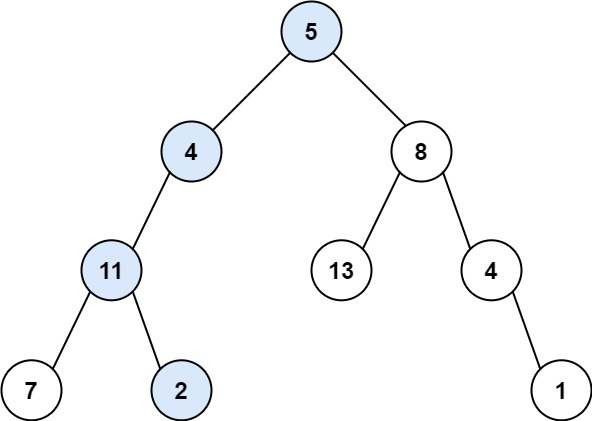``` Input: root = [5,4,8,11,null,13,4,7,2,null,null,null,1], targetSum = 22 Output: true ```

Example 2:``` Input: root = [1,2,3], targetSum = 5 Output: false ```

Example 3:

``` Input: root = [1,2], targetSum = 0 Output: false ```

2. Has Path Sum – Solution

The following is a solution which demonstrates how to determine if a binary tree root to leaf path sum exists.

``` 2. Has Path Sum - Solution C# // ============================================================================ // Author: Kenneth Perkins // Date: Oct 18, 2021 // Taken From: http://programmingnotes.org/ // File: Solution.cs // Description: Determines if a root to leaf path sum exists in a binary tree // ============================================================================ /** * Definition for a binary tree node. * public class TreeNode { * public int val; * public TreeNode left; * public TreeNode right; * public TreeNode(int val=0, TreeNode left=null, TreeNode right=null) { * this.val = val; * this.left = left; * this.right = right; * } * } */ public class Solution { public bool HasPathSum(TreeNode root, int targetSum) { return Search(root, targetSum); } public bool Search(TreeNode node, int targetSum) { if (node == null) { return false; } // Determine the current sum targetSum = targetSum - node.val; // Since this is a root-to-leaf check, evaluate for the // success condition when both left and right nodes are null if (node.left == null && node.right == null) { return targetSum == 0; } // Keep exploring along branches finding the target sum return Search(node.left, targetSum) || Search(node.right, targetSum); } }// http://programmingnotes.org/ 1234567891011121314151617181920212223242526272829303132333435363738394041424344 // ============================================================================//    Author: Kenneth Perkins//    Date:   Oct 18, 2021//    Taken From: http://programmingnotes.org///    File:  Solution.cs//    Description: Determines if a root to leaf path sum exists in a binary tree// ============================================================================/** * Definition for a binary tree node. * public class TreeNode { *     public int val; *     public TreeNode left; *     public TreeNode right; *     public TreeNode(int val=0, TreeNode left=null, TreeNode right=null) { *         this.val = val; *         this.left = left; *         this.right = right; *     } * } */public class Solution {    public bool HasPathSum(TreeNode root, int targetSum) {        return Search(root, targetSum);    }     public bool Search(TreeNode node, int targetSum) {        if (node == null) {            return false;        }         // Determine the current sum        targetSum = targetSum - node.val;         // Since this is a root-to-leaf check, evaluate for the        // success condition when both left and right nodes are null        if (node.left == null && node.right == null) {            return targetSum == 0;        }         // Keep exploring along branches finding the target sum        return Search(node.left, targetSum)            || Search(node.right, targetSum);    }}// http://programmingnotes.org/ ```

QUICK NOTES:
The highlighted lines are sections of interest to look out for.

The code is heavily commented, so no further insight is necessary. If you have any questions, feel free to leave a comment below.

Once compiled, you should get this as your output for the example cases:

``` true false false ```

## C# || How To Convert Sorted Array to Binary Search Tree Using C#The following is a module with functions which demonstrates how to convert a sorted array to a binary search tree using C#.

1. Sorted Array To BST – Problem Statement

Given an integer array nums where the elements are sorted in ascending order, convert it to a height-balanced binary search tree.

A height-balanced binary tree is a binary tree in which the depth of the two subtrees of every node never differs by more than one.

Example 1: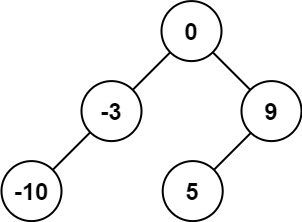``` Input: nums = [-10,-3,0,5,9] Output: [0,-3,9,-10,null,5] Explanation: [0,-10,5,null,-3,null,9] is also accepted: ```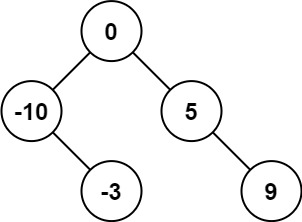Example 2: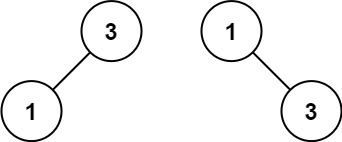``` Input: nums = [1,3] Output: [3,1] Explanation: [1,3] and [3,1] are both a height-balanced BSTs. ```

2. Sorted Array To BST – Solution

The following is a solution which demonstrates how to convert a sorted array to a binary search tree.

``` 2. Sorted Array To BST - Solution C# // ============================================================================ // Author: Kenneth Perkins // Date: Oct 18, 2021 // Taken From: http://programmingnotes.org/ // File: Solution.cs // Description: Demonstrates how to convert sorted array to BST // ============================================================================ /** * Definition for a binary tree node. * public class TreeNode { * public int val; * public TreeNode left; * public TreeNode right; * public TreeNode(int val=0, TreeNode left=null, TreeNode right=null) { * this.val = val; * this.left = left; * this.right = right; * } * } */ public class Solution { public TreeNode SortedArrayToBST(int[] nums) { return BuildBST(nums, 0, nums.Length -1); } TreeNode BuildBST(int[] nums, int start, int end) { if (start > end) { return null; } var mid = start + (end - start) / 2; var node = new TreeNode(nums[mid]); node.left = BuildBST(nums, start, mid - 1); node.right = BuildBST(nums, mid + 1, end); return node; } }// http://programmingnotes.org/ 12345678910111213141516171819202122232425262728293031323334353637 // ============================================================================//    Author: Kenneth Perkins//    Date:   Oct 18, 2021//    Taken From: http://programmingnotes.org///    File:  Solution.cs//    Description: Demonstrates how to convert sorted array to BST// ============================================================================/** * Definition for a binary tree node. * public class TreeNode { *     public int val; *     public TreeNode left; *     public TreeNode right; *     public TreeNode(int val=0, TreeNode left=null, TreeNode right=null) { *         this.val = val; *         this.left = left; *         this.right = right; *     } * } */public class Solution {    public TreeNode SortedArrayToBST(int[] nums) {        return BuildBST(nums, 0, nums.Length -1);    }     TreeNode BuildBST(int[] nums, int start, int end) {        if (start > end) {            return null;        }         var mid = start + (end - start) / 2;        var node = new TreeNode(nums[mid]);        node.left = BuildBST(nums, start, mid - 1);        node.right = BuildBST(nums, mid + 1, end);        return node;    }}// http://programmingnotes.org/ ```

QUICK NOTES:
The highlighted lines are sections of interest to look out for.

The code is heavily commented, so no further insight is necessary. If you have any questions, feel free to leave a comment below.

Once compiled, you should get this as your output for the example cases:

``` [0,-10,5,null,-3,null,9] [1,null,3] ```

## C# || How To Convert 1D Array Into 2D Array Using C#The following is a module with functions which demonstrates how to convert a 1D array into a 2D array using C#.

1. Construct 2D Array – Problem Statement

You are given a 0-indexed 1-dimensional (1D) integer array original, and two integers, m and n. You are tasked with creating a 2-dimensional (2D) array with m rows and n columns using all the elements from original.

The elements from indices 0 to n – 1 (inclusive) of original should form the first row of the constructed 2D array, the elements from indices n to 2 * n – 1 (inclusive) should form the second row of the constructed 2D array, and so on.

Return an m x n 2D array constructed according to the above procedure, or an empty 2D array if it is impossible.

Example 1: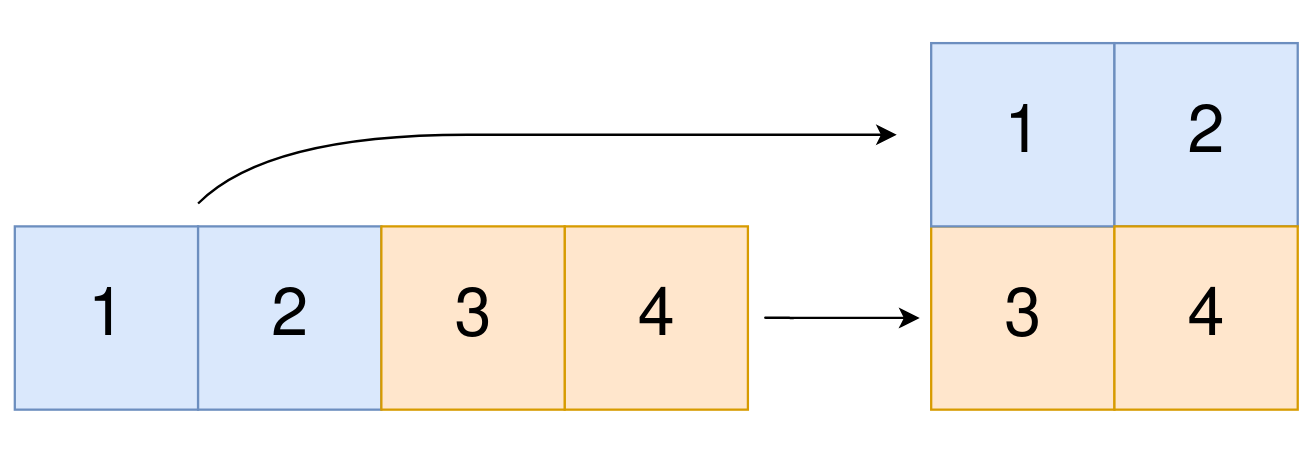``` Input: original = [1,2,3,4], m = 2, n = 2 Output: [[1,2],[3,4]] Explanation: The constructed 2D array should contain 2 rows and 2 columns. The first group of n=2 elements in original, [1,2], becomes the first row in the constructed 2D array. The second group of n=2 elements in original, [3,4], becomes the second row in the constructed 2D array. ```

Example 2:

``` Input: original = [1,2,3], m = 1, n = 3 Output: [[1,2,3]] Explanation: The constructed 2D array should contain 1 row and 3 columns. Put all three elements in original into the first row of the constructed 2D array. ```

Example 3:

``` Input: original = [1,2], m = 1, n = 1 Output: [] Explanation: There are 2 elements in original. It is impossible to fit 2 elements in a 1x1 2D array, so return an empty 2D array. ```

Example 4:

``` Input: original = , m = 1, n = 2 Output: [] Explanation: There is 1 element in original. It is impossible to make 1 element fill all the spots in a 1x2 2D array, so return an empty 2D array. ```

2. Construct 2D Array – Solution

The following is a solution which demonstrates how to convert a 1D array into a 2D array.

``` 2. Construct 2D Array - Solution C# // ============================================================================ // Author: Kenneth Perkins // Date: Oct 18, 2021 // Taken From: http://programmingnotes.org/ // File: Solution.cs // Description: Demonstrates how to convert a 1D array into a 2D array // ============================================================================ public class Solution { public int[][] Construct2DArray(int[] original, int m, int n) { // Determine if parameters are valid if (original.Length != m * n) { return new int[]; } // Create 2D array var result = new int[m][]; for (int row = 0; row < result.Length; ++row) { result[row] = new int[n]; } // Populate result for (int index = 0; index < original.Length; ++index) { // Determine the result array row and column index var rowIndex = index / n; var colIndex = index % n; // Copy values to result result[rowIndex][colIndex] = original[index]; } return result; } }// http://programmingnotes.org/ 123456789101112131415161718192021222324252627282930313233 // ============================================================================//    Author: Kenneth Perkins//    Date:   Oct 18, 2021//    Taken From: http://programmingnotes.org///    File:  Solution.cs//    Description: Demonstrates how to convert a 1D array into a 2D array// ============================================================================public class Solution {    public int[][] Construct2DArray(int[] original, int m, int n) {        // Determine if parameters are valid        if (original.Length != m * n) {            return new int[];        }         // Create 2D array        var result = new int[m][];        for (int row = 0; row < result.Length; ++row) {            result[row] = new int[n];        }         // Populate result        for (int index = 0; index < original.Length; ++index) {            // Determine the result array row and column index            var rowIndex = index / n;            var colIndex = index % n;             // Copy values to result            result[rowIndex][colIndex] = original[index];        }         return result;    }}// http://programmingnotes.org/ ```

QUICK NOTES:
The highlighted lines are sections of interest to look out for.

The code is heavily commented, so no further insight is necessary. If you have any questions, feel free to leave a comment below.

Once compiled, you should get this as your output for the example cases:

``` [[1,2],[3,4]] [[1,2,3]] [] [] ```